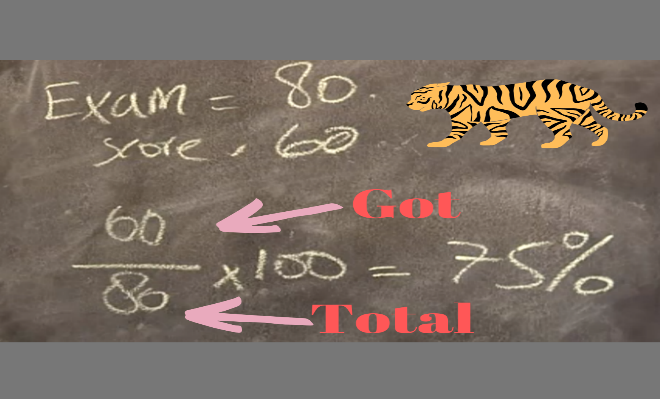# How To Calculate Percentage – Many ways

Knowing how to calculate a percentage will not only help you get good grades on math tests but in the real world as well. It is used to calculate tips at restaurants, find the nutritional content of your food or figure out your favorite sports team.

Regardless of how you plan to use the knowledge, percentage calculation is basic and overall quite simple.

History: >>> Percent symbol use since 15th Century

## What is The Percentage?

Percentage means out every 100 what outcomes got. (%) Symbol as a quick way to register fractions with 100 nominal values.

For example, it 12 days holiday out of every 100 days,” “it says 12% holiday of the time.”

## The Formula For Calculating Percentages

### Here you can easy way to understand

PERCENTAGE = GOT / TOTAL X 100

You can see example how to calculate percentage, your children obtain 60 marks out of 80 if you convert it into percentage it will be 75%.### Examples of percentage calculations

The following three examples show how to calculate percentages.

1. 25 people out of a total of 50 were male. What percentage were male?23 / 50 * 100 = 46%

2. The price of a \$500 mobile is increased by 25%. What will the new price?Step 1) \$500 * 25/100 = \$125

Step 2) \$500 + \$125 = \$625

3. The tax on an item is \$6.00. The tax rate is 15%. What is the price without tax?Step: 1) P * 25/100 = 8

Step: 2) P * 25/100 * 100 = 8*100

Step: 3) P = 8*100/25

Price without tax is = \$32

## Tips: How to find out percentage easy way

A best way of finding percentages is to start by finding what 1% is any number.

Example: What is 12.5% of 43?

1st step: Find out 1% of 43

43/100 = 0.43

2nd Step  Now we have 1% of 43 = 0.43, We just need to multiply it by 12.5 to find 12.5% .

12.5*0.43=5.375

Answer: 12.5% of 43 is 5.375

Now you can calculate any giant amount easy way by using this trick.